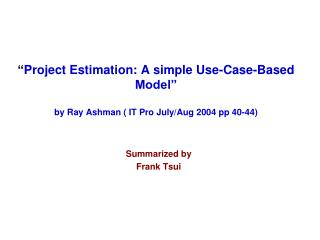DownloadDownload PresentationSummarized by Frank Tsui

# Summarized by Frank Tsui

Télécharger la présentation## Summarized by Frank Tsui

- - - - - - - - - - - - - - - - - - - - - - - - - - - E N D - - - - - - - - - - - - - - - - - - - - - - - - - - -
##### Presentation Transcript

1. “Project Estimation: A simple Use-Case-Based Model” by Ray Ashman ( IT Pro July/Aug 2004 pp 40-44) Summarized by Frank Tsui

2. From Chaos Report we know that cost overrun can range from less than 20% to over 400%, with average cost over-run for failed projects: 178% for large companies 182% for medium companies 214% for small companies Project estimation is a difficult task ---- why not use the pastdata to help in the estimation task. ** This article proposes a technique of project effort estimation based on iterative development process, comparing and improving successive iterations of project effort estimates with the actual data.** • Article assumes you, as a software manager, have a feel for: • iterative dev. process and • Use-Case methodology

3. On Measurement and Initial Model • “Scheduled measurement” • Understood measurements of: total cost or total resources • “Unscheduled measurement” • Not so well understood areas such as: rework, requirements changes, people risk, technology changes. • Time unit as the basis for measurement • Effort and cost must include the time parameter in a form of a “rate” measurement. • e.g. 150 person-months as effort is not enough; also show assumed productivity rate of 30FP/person-month or 770 loc/person-month - Author’s calling “time” as type of measurement is misguided ! - Author included these 3 thoughts in creating a 1st-cut model for cost estimation (next slide)

4. Iteratively “Improve” the Cost-Estimation Model Identify the metric Create “model” Generate estimate Refine metrics Compare with actual Output estimate Start with a simple approach and iteratively refine the metrics for estimation.

5. Utilize Requirements (Use-Cases) as the Basisfor Cost Estimation • Requirements clearly should be a key inputto cost (effort)estimation • But requirements are represented in many different ways and may be carried to different “depth” level. • Use-caseprovides a certain amount of consistency in the way requirements are • captured, • analyzed (priority, risks, complexity, difficulty, etc.) and • expressed • Also advocates iterative developmentprocess and use a “scalefactor”to improve the estimate. Perform Effort-Estimation for each set of Use-Case and Iteratively Improve

6. Figure 4 • Have you figured how it works?

7. Methodology: Estimating by Use-Cases (Reqs) by Iterations and including the Actuals (assumes inclusion of analysis of many factors such as priority, risk, complexity, multiple sources, etc.. in the estimate here.) Accum. Actual total Initial Effort est. Actual (2nd iter.) Actual (3rd iter.) Actual (1st iter.) Requirements (by Use-Case) use-case 1 5 pd 6 pd use-case 2 8 9 use-case 3 7 6 use-case 4 10 12 use-case 5 5 5 (Estimated) Total 35pd Scaling Factor 15/13=1.15 21/20=1.05 38/35=1.08 “New” estimate 35x1.15=40.3 “Newer” 35x1.05=36.8 “Newest”35x1.08=37.8 15 21 38

8. Author’s “model” aims are: • to be able to capture the estimator’s experience • and • b) to increase the accuracy of successive estimates based on “repeatable measurements”

9. The Underlying Theory on Estimations(originally from B. Boehm) 4 x N Actual N .25 x N Req Des code Test Ship